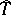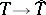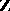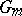# Algebraic torus

(diff) ← Older revision | Latest revision (diff) | Newer revision → (diff)
An algebraic group that is isomorphic over some extension of the ground field to the direct product of a finite number of multiplicative groups. The groupof all algebraic homomorphisms of an algebraic torusinis known as the character group of; it is a free Abelian group of a rank equal to the dimension of. If the algebraic torusis defined over a field, thenhas a-module structure, whereis the Galois group of the separable closure of. The functordefines a duality between the category of algebraic tori overand the category of-free-modules of finite rank. An algebraic torus overthat is isomorphic to a product of groupsover its ground fieldis called split over; any algebraic torus oversplits over a finite separable extension of. The role played by algebraic tori in the theory of algebraic groups greatly resembles the role played by tori in the theory of Lie groups. The study of algebraic tori defined over algebraic number fields and other fields, such as finite fields, occupies an important place in problems of arithmetic and in the classification of algebraic groups. Cf. Linear algebraic group; Tamagawa number.